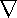# Maths - Grad

Grad is short for gradient, it takes a scalar field as input and returns a vector field, for a 3 dimensional vector field it is defined as follows:

 grad(s) = i ∂s + j ∂s + k ∂s ∂x ∂y ∂z

where,

• i,j and k are unit vectors representing the axis of the Cartesian coordinates.
• s is the scalar field, i.e. a scalar value which is a function of its position.

Or in more conventional vector notation:

 ∂s ∂x
 ∂s ∂y
 ∂s ∂z

The vector returned has a direction which shows the greatest rate of change and its magnitude |v| tells us what that rate of change is. If you apply a grad to, say the height of a hill, you will get a vector pointing directly uphill, the length of which tells you how steep the hill is. Perhaps that may not be a good example as the height is normally the third dimension in a position vector but here we are separating it out as a scalar on a 2 dimensional map. Another example might be temperature, which is a scalar quantity, which might vary over 3 dimensions. grad of this temperature field would show where the temperature is changing most rapidly and would give the direction of heat flow.

If n is a normal to the contour lines then

 grad(s) = ∂s n ∂n

where:

n = normal vector

If the contour lines are closer together then grad(s) will be greater.

## Vector Operator

The 'Del' operator represented by the 'Nabla' symbolis used to represent the vector operator, as follows:= i ∂ + j ∂ + k ∂ ∂x ∂y ∂z

or in more conventional vector notation:=
 ∂ ∂x
 ∂ ∂y
 ∂ ∂z

The del symbol does not have any meaning on its own, only when applied to a scalar field. Or a vector field as shown later.s = grad(s)

## Sum of Scalar Fields

Adding the scalar fields is equivalent to adding the grad functions:

In the diagram below we have plotted the potential energy (scalar) field for:

1. a single mass at x=-3
2. a single mass at x=+3
3. two masses at x=-3 and x=+3.how this plot was produced.

As we can see the field for the two masses is the sum of the other two fields.

## Identities

sum of scalar fields:

product of scalar fields:

grad of dot product of vector fields:

grad(v1v2) = (v2) v1 + (v1) v2 + v2 × curl(v1) +v1 × curl(v2)

where:

• v1, v2 = vectors
•= del operator
• • = vector dot product
• × = vector cross product
• curl() = curl function

 metadata block see also: Vectors Correspondence about this page Book Shop - Further reading. Where I can, I have put links to Amazon for books that are relevant to the subject, click on the appropriate country flag to get more details of the book or to buy it from them. Other Math Books Commercial Software Shop Where I can, I have put links to Amazon for commercial software, not directly related to the software project, but related to the subject being discussed, click on the appropriate country flag to get more details of the software or to buy it from them.

This site may have errors. Don't use for critical systems.